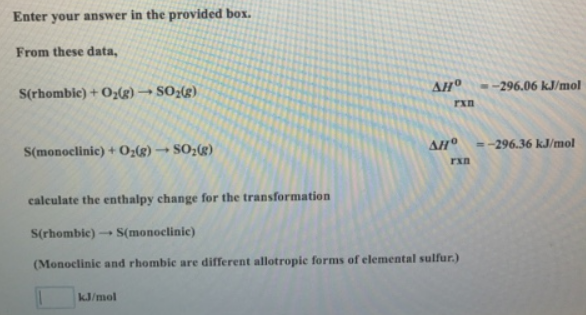# Problem: Enter your answer in the provided box.From these data, S (rhombic) + O2 (g) → SO2 (g)            ΔH°rxn = -296.06 kJ/mol S (monoclinic) + O2 (g) → SO2 (g)       ΔH°rxn = - 296.36 kJ/mol calculate the enthalpy change for the transformation S (rhombic) → S (monoclinic) (Monoclinic and rhombic are different allotropic forms of elemental sulfur.)

###### FREE Expert Solution
96% (356 ratings)###### Problem Details

Enter your answer in the provided box.

From these data,

S (rhombic) + O2 (g) → SO2 (g)            ΔH°rxn = -296.06 kJ/mol

S (monoclinic) + O2 (g) → SO2 (g)       ΔH°rxn = - 296.36 kJ/mol

calculate the enthalpy change for the transformation

S (rhombic) → S (monoclinic)

(Monoclinic and rhombic are different allotropic forms of elemental sulfur.)Frequently Asked Questions

What scientific concept do you need to know in order to solve this problem?

Our tutors have indicated that to solve this problem you will need to apply the Hess's Law concept. You can view video lessons to learn Hess's Law. Or if you need more Hess's Law practice, you can also practice Hess's Law practice problems.

What professor is this problem relevant for?

Based on our data, we think this problem is relevant for Professor Buckley's class at WSU.